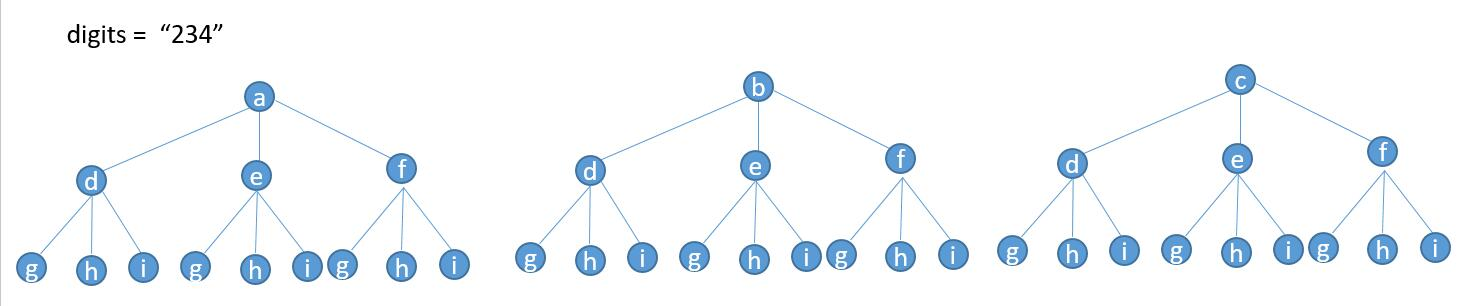# 4 sums and letter combinations of a phone number

Let’s continue…

Problem Statement:

4 Sums

Given an array `nums` of n integers and an integer `target`, are there elements a, b, c, and d in `nums` such that a + b + c + d = `target`? Find all unique quadruplets in the array which gives the sum of `target`.

Note:

The solution set must not contain duplicate quadruplets.

Example:

```Given array nums = [1, 0, -1, 0, -2, 2], and target = 0.

A solution set is:
[
[-1,  0, 0, 1],
[-2, -1, 1, 2],
[-2,  0, 0, 2]
]
```

Solution:

Basically, the idea is the same as 3 sums solution two. In 3 Sums problems, we first fix one of the numbers, and we use two pointers to keep track of the rest of the two numbers. Basically, in this problem, we first fix one number, and then we use three pointers to keep track of the rest three numbers. In general, this method will provide a relative slow but decent solution.

Time Complexity: O(n^3)

Space Complexity: O(n) The worst case could be that all the permutations of the elements.

This file contains bidirectional Unicode text that may be interpreted or compiled differently than what appears below. To review, open the file in an editor that reveals hidden Unicode characters.

 class Solution { public: vector> fourSum(vector& nums, int target) { vector> finalResult; int len = nums.size(); if(len <= 3) return finalResult; else sort(nums.begin(), nums.end()); for(int i = 0; i< len – 3😉 { int a = nums[i]; for(int j = i+1; j < len – 2😉 { int b = nums[j]; for(int p = j + 1, q = len-1; p < q;) { int c = nums[p], d = nums[q]; int value = a + b + c + d; if(value == target) { finalResult.push_back({a,b,c,d}); while(d == nums[–q] ); while(c == nums[++p]); } else if(value > target){ while(d == nums[–q] ); } else{ while(c == nums[++p]); } } j++; while(nums[j-1] == nums[j]&& j

view raw

4 sums.cpp

hosted with ❤ by GitHub

Problem Statement:

Letter combinations of a phone number

Given a string containing digits from `2-9` inclusive, return all possible letter combinations that the number could represent.

A mapping of digit to letters (just like on the telephone buttons) is given below. Note that 1 does not map to any letters.Example:

```Input: "23"
Output: ["ad", "ae", "af", "bd", "be", "bf", "cd", "ce", "cf"].
```

Note:

Although the above answer is in lexicographical order, your answer could be in any order you want.

Solution One: Depth First Searching

When we want to solve problem related to permutation, we first need to draw a graph like below:Let’s say if we want to determine all possible outcomes from a string digit “234”, then the picture above could show all the possible permutations. We can see from the graph, if we want to find the possible outcomes, we can simply iterate from the top to the bottom, and when we reach the bottom, we push the result into a our final string vector. When we are done with one path (from the top to the bottom), we switch to another path and do the same thing.

What does it look like? A depth first searching algorithm! That’s indeed what we are going to implement.

Time Complexity: approximately O(3^n) .n is the number of digit numbers.

Space Complexity: approximately O(n*3^n). We have approximately 3^n paths, with each path we will have n nodes.

This file contains bidirectional Unicode text that may be interpreted or compiled differently than what appears below. To review, open the file in an editor that reveals hidden Unicode characters.

 class Solution { private: vector dic_s = {"", "", "abc", "def", "ghi","jkl", "mno","pqrs", "tuv", "wxyz"}; vector result; public: void dfs(const string &digits, string s, int pos){ int len = digits.size(); if(pos >= len){ return; } int subLen = dic_s[digits[pos] – '0'].size(); for(int i = 0; i< subLen; i++) { string dic_string = dic_s[digits[pos] – '0']; string temp = s + dic_string[i]; if(pos == len – 1) { result.push_back(temp); } dfs(digits, temp, pos + 1); } } vector letterCombinations(string digits) { dfs(digits, "", 0); return result; } };

Solution Two: String multiplication

The idea comes from here.

The logic here is simple. Whenever we encounter digit numbers, we treat it as a string list. For example, if the digit number is “23”, then we treat this number as [‘a’ , ‘b’ , ‘c’] and [‘d’ , ‘e’ , ‘f’]. Then we can write two for loops to generate the permutation of their result. Let me call this function vector<string> multiStr(vector l1, vector l2), where l2 is the string list of a new parsed digit number, l1 is the answer up to the previous digit number.

Then the idea will be simple, we parse each digit number, and call the multiStr() function to calculate the new result, and then continue until the last digit number.

Time Complexity: Approximately O(3^n) Note the outer for loop in multStr() function gets more and more expensive. n is the number of digit numbers.

Space Complexity: Approximately O(3^n) Note that the auxiliary vector becomes more and more expensive.

This file contains bidirectional Unicode text that may be interpreted or compiled differently than what appears below. To review, open the file in an editor that reveals hidden Unicode characters.